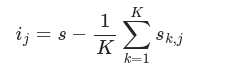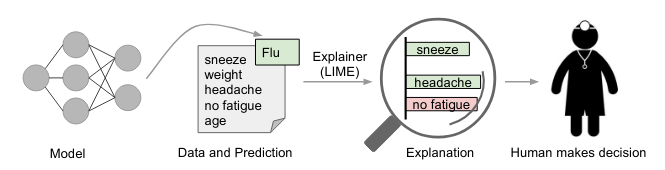This article was published as a part of the Data Science Blogathon

## eXplainable AI(XAI)

What does Interpretability/Explainability mean in AI?

The following points encompass the explainability-

• How the internal working of the so-called black box(machine/deep learning models) can be interpreted/explained in human terms
• In addition to the outcome from our model if we know why it has arrived at such output i.e output backed by proper explanation, will be good to drive the business
• How can we build trust in such black box models?
• Scope:Local(per sample) or Global(overall) interpretation

Algorithms such as linear/logistic regression are easy to interpret based on model coefficients and Tree-based algorithms which can help us understand how it’s making decisions, with built-in support for feature importance and visualization.

## What about complex Machine learning algorithms?

### Permutation Feature Importance

One simple method is Permutation Feature Importance, It is a model inspection technique that can be used for any fitted estimator when the data is tabular. The permutation feature importance is defined to be the decrease in a model score when a single feature value is randomly shuffled. This procedure breaks the relationship between the feature and the target, thus the drop in the model score is indicative of how much the model depends on the feature.

#### Algorithm

• Train a model on a dataset
• Calculate the error metrics or score(s) for the trained model for reference
• Shuffled the validation data columns values one column(features) at a time and can be repeated K times
• Using the shuffled data to evaluate the trained model using the same error or score metrics for each iteration
• The features that affect the error metrics most are the important ones as it indicates a model dependency on that features
• We can calculate the importance score for each feature asWhere,

ij is the feature importance of feature j

s is the reference score calculated from the trained model

K is the number of iterations(shuffling operations) performed for a feature

sk,j is the score for kth iteration on feature j

We can use the python sklearn package for build-in permutation_importance function

`from sklearn.inspection import permutation_importance`

We will be using the Xgboost algorithm with default parameters on the Boston dataset

```xg = xgboost.XGBRegressor()
xg.fit(Xtrain, ytrain)```

Now we will use the permutation_importance function on the test set to calculate the feature importance, we need to provide a trained model and number of shuffling iteration to perform (n_repeats parameter)

```r = permutation_importance(xg, Xtest, ytest,
n_repeats=30,
random_state=0)```

Here, we have set n_repeats=30

The returned object, r will contain feature importance values for each feature which we can visualize using the matplotlib python library

```plt.figure(figsize=(10,4))
plt.bar(boston.feature_names,r.importances_mean)
plt.xlabel('Features')
plt.ylabel('Mean Importance')
plt.title('Feature importance using Feature Permutation Importance');```

Source: Github

## Caveats

If two features are correlated and one of them is permuted then

• The model still has the other correlated feature and in that case, both features will have lower importance value even if those are actually important
• It can be biased by unrealistic data instances formed by permutation

Computationally expensive for a large number of features

A good practice is to drop one of the correlated features based on domain understanding and try to apply the Permutation Feature Importance algorithm which will provide better feature understanding.

Let’s discuss another method to interpret the black box models.

## Global surrogate models

• An interpretation model trained to approximate the predictions of the black-box models
• It’s like solving black box Interpretability task using simpler and explainable models (such as linear regression, decision tree,..) i.e explaining machine learning using more machine learning

## How does it work?

• Get the predictions(yhat) from the black-box model
• Select any simple and explainable model(linear reg., decision tree..) as per the use case
• Train the selected model on the same dataset used for training the black-box model, using predictions(yhat) as the target
• Measure the performance, as to how well the surrogate model approximates the behavior of the black-box model
• Finally, we can interpret the global surrogate model

Flexible, as a selection of surrogate model, does not depend on the black-box model. If at some time we have a better performing black-box model in place of an existing black box, we do not have to change the method of interpretation

▪️How much confidence is enough(% of variance explained) in deciding the surrogate model is close enough to the black-box model
▪️ Interpretation becomes irrelevant if the black-box model is not performing well

## LIME (Local Interpretation Model agnostic Explanation)

We have seen few model interpretation techniques for Global interpretation, what about Local Interpretation i.e when we want to understand how a model prediction was made for a particular observation.

Consider a loan approval model, what if a user request is declined, then the user has the right to question WHY? and authorities should know why the model has declined the user request and communicate the same to the user as they just can’t say that their system has rejected it instead they need to explain on what factors(features) the request is rejected.

▪️LIME can explain the predictions of any classifier or regressor in a faithful way, by approximating it locally with an interpretable model(linear reg., decision tree..)

▪️It tests what happens to the predictions when we feed variations of the data into the machine learning model

▪️Can be used on tabular, text, and image dataSource: LIME Paper

We can use python lime library to interpret models

```import lime
import lime.lime_tabular```

We will be using the Xgboost algorithm with default parameters on the Boston dataset

```xg = xgboost.XGBRegressor()
xg.fit(Xtrain, ytrain)```

Now we will create a lime explainer object, for which we have to specify the target column, features names, categorical features, and mode of the algorithm(regression or classification)

```explainer = lime.lime_tabular.LimeTabularExplainer(Xtrain,
feature_names=boston.feature_names,
class_names=['price'],
categorical_features=categorical_features,
verbose=True, mode='regression')```

We can use the explain_instance method of the explainer object to interpret a particular instance of data

`exp = explainer.explain_instance(Xtest[i], xg.predict, num_features=5)`

i is the index in test data that we need to interpret

we can visualize the interpretation output using the show_in_notebook method

`exp.show_in_notebook(show_table=True)`

We will get similar to below output

Source: Github

For Complete code refer to Github Repository

## References

https://christophm.github.io/interpretable-ml-book/feature-importance.html

https://christophm.github.io/interpretable-ml-book/global.html

https://scikit-learn.org/stable/modules/permutation_importance.html

https://scikit-learn.org/stable/auto_examples/inspection/plot_permutation_importance_multicollinear.html#sphx-glr-auto-examples-inspection-plot-permutation-importance-multicollinear-py

LIME Research Paper: https://arxiv.org/pdf/1602.04938.pdf

https://github.com/marcotcr/lime